ALL

Page: (Previous)   1  2  3  4  5  (Next)
ALL

### M

#### MAT-HS.A-CED.01.a

 MAT-HS Targeted Standards(A) Concept: Algebra(CED) Domain: Creating ExpressionsCluster: Create equations that describe numbers or relationships MAT-HS.A-CED.01.a Create and solve linear equations in one variable.

## Student Learning Targets:

• I can

• I can

### Skills (Performance) Targets

• I can create a linear equation or inequality given a situation or set of data.

• I can

## Proficiency Scale

Score   Description Sample Activity
4.0 In addition to achieving level 3.0 content, the student makes in-depth inferences and applications that go beyond what was taught
• Write an equation of a parabola that has two x-intercepts and a minimum vertex.

• Create a rational equation given a situation or set or data
3.5 In addition to Score 3.0 performance, the student demonstrates in-depth inferences and applications regarding the more complex content with partial success.
3.0

Can create equations and inequalities in one variable and use them to solve problems (linear only)

The student exhibits no major errors or omissions.
• An online music club sells compact discs for $13.95 each plus$1.95 shipping and handling per order.  Maria’s total bill was $85.65. Create an equation to model this situation. Solve your equation to determine how many compact discs were sold. • Suppose you are working for a trucking company. Your job is to load a truck with at least 5000 lb of freight. You have loaded 2395 lb of freight, but you have to unload 50 lb that was loaded by mistake. Write and solve an inequality to find how many more pounds you need to load. • Which kind of function best models the data in the table? Write an equation to model the data.  x y 0 5 1 7 2 9 3 11 2.5 The student demonstrates no major errors or omissions regarding the simpler details and processes (Score 2.0 content) and partial knowledge of the more complex ideas and processes (Score 3.0 content). 2.0 There are no major errors or omissions regarding the simpler details and processes as the student: • recognizes and recalls specific terminology, such as: (A.SSE.1) • linear • correctly identifies whether the relationship is linear or exponential. • writes the general equations for linear and exponential functions. • pick out relevant information in a contextual problem. However, the student exhibits major errors or omissions regarding the more complex ideas and processes. - 1.5 The student demonstrates partial knowledge of the simpler details and processes (Score 2.0 content) but exhibits major errors or omissions regarding the more complex ideas and procedures (Score 3.0 content). 1.0 With help, a partial understanding of some of the simpler details and processes and some of the more complex ideas and processes. - 0.5 With help, the student demonstrates a partial understanding of some of the simpler details and processes (Score 2.0 content) but not the more complex ideas and processes (Score 3.0 content). ## Resources  Web Vocab linear equations quadratic equations rational equations exponential equations inequalities #### MAT-HS.A-CED.01.b  MAT-HS Targeted Standards(A) Concept: Algebra(CED) Domain: Creating ExpressionsCluster: Create equations that describe numbers or relationships MAT-HS.A-CED.01.b Create and solve exponential equations in one variable ## Student Learning Targets: ### Knowledge Targets • I can ### Reasoning Targets • I can ### Skills (Performance) Targets • I can ### Product Targets • I can ## Proficiency Scale  Score Description Sample Activity 4.0 (advanced) In addition to Score 3.0, the student demonstrates in-depth inferences and applications regarding more complex material that go beyond end of instruction expectations. 3.5 In addition to Score 3.0 performance, the student demonstrates in-depth inferences and applications regarding the more complex content with partial success. 3.0 (proficient) The student can: The student exhibits no major errors or omissions. 2.5 The student demonstrates no major errors or omissions regarding the simpler details and processes (Score 2.0 content) and partial knowledge of the more complex ideas and processes (Score 3.0 content). 2.0 (progressing) There are no major errors or omissions regarding the simpler details and processes as the student: However, the student exhibits major errors or omissions regarding the more complex ideas and processes. 1.5 The student demonstrates partial knowledge of the simpler details and processes (Score 2.0 content) but exhibits major errors or omissions regarding the more complex ideas and procedures (Score 3.0 content). 1.0 (beginning) With help, the student demonstrates a partial understanding of some of the simpler details and processes (Score 2.0 content) and some of the more complex ideas and processes (Score 3.0 content). - 0.5 With help, the student demonstrates a partial understanding of some of the simpler details and processes (Score 2.0 content) but not the more complex ideas and processes (Score 3.0 content). 0.0 Even with help, the student demonstrates no understanding or skill. - ## Resources  Web Vocab #### MAT-HS.A-CED.01.c  MAT-HS Targeted Standards(A) Concept: Algebra(CED) Domain: Creating ExpressionsCluster: Create equations that describe numbers or relationships MAT-HS.A-CED.01.c Create and solve linear inequalities in one variable. ## Student Learning Targets: ### Knowledge Targets • I can ### Reasoning Targets • I can • I can ### Skills (Performance) Targets • I can create a linear equation or inequality given a situation or set of data. ### Product Targets • I can ## Proficiency Scale Score Description Sample Activity 4.0 In addition to achieving level 3.0 content, the student makes in-depth inferences and applications that go beyond what was taught • Write an equation of a parabola that has two x-intercepts and a minimum vertex. • Create a rational equation given a situation or set or data 3.5 In addition to Score 3.0 performance, the student demonstrates in-depth inferences and applications regarding the more complex content with partial success. 3.0 Can create equations and inequalities in one variable and use them to solve problems (linear only) The student exhibits no major errors or omissions. • An online music club sells compact discs for$13.95 each plus $1.95 shipping and handling per order. Maria’s total bill was$85.65.  Create an equation to model this situation.  Solve your equation to determine how many compact discs were sold.

• Suppose you are working for a trucking company. Your job is to load a truck with at least 5000 lb of freight. You have loaded 2395 lb of freight, but you have to unload 50 lb that was loaded by mistake. Write and solve an inequality to find how many more pounds you need to load.

• Which kind of function best models the data in the table? Write an equation to model the data.

 x y 0 5 1 7 2 9 3 11
2.5 The student demonstrates no major errors or omissions regarding the simpler details and processes (Score 2.0 content) and partial knowledge of the more complex ideas and processes (Score 3.0 content).
2.0

There are no major errors or omissions regarding the simpler details and processes as the student:

• recognizes and recalls specific terminology, such as: (A.SSE.1)

• linear

• correctly identifies whether the relationship is linear or  exponential.

• writes the general equations for linear and exponential functions.

• pick out relevant information in a contextual problem.

However, the student exhibits major errors or omissions regarding the more complex ideas and processes.
-
1.5 The student demonstrates partial knowledge of the simpler details and processes (Score 2.0 content) but exhibits major errors or omissions regarding the more complex ideas and procedures (Score 3.0 content).
1.0 With help, a partial understanding of some of the simpler details and processes and some of the more complex ideas and processes. -
0.5 With help, the student demonstrates a partial understanding of some of the simpler details and processes (Score 2.0 content) but not the more complex ideas and processes (Score 3.0 content).

## Resources

 Web Vocab linear equations quadratic equations rational equations exponential equations inequalities

#### MAT-HS.A-CED.01.d

 MAT-HS Targeted Standards(A) Concept: Algebra(CED) Domain: Creating ExpressionsCluster: Create equations that describe numbers or relationships MAT-HS.A-CED.01.d Create and solve exponential inequalities in one variable

• I can

• I can

• I can

• I can

## Proficiency Scale

 Score Description Sample Activity 4.0 (advanced) In addition to Score 3.0, the student demonstrates in-depth inferences and applications regarding more complex material that go beyond end of instruction expectations. 3.5 In addition to Score 3.0 performance, the student demonstrates in-depth inferences and applications regarding the more complex content with partial success. 3.0 (proficient) The student can: The student exhibits no major errors or omissions. 2.5 The student demonstrates no major errors or omissions regarding the simpler details and processes (Score 2.0 content) and partial knowledge of the more complex ideas and processes (Score 3.0 content). 2.0 (progressing) There are no major errors or omissions regarding the simpler details and processes as the student:   However, the student exhibits major errors or omissions regarding the more complex ideas and processes. 1.5 The student demonstrates partial knowledge of the simpler details and processes (Score 2.0 content) but exhibits major errors or omissions regarding the more complex ideas and procedures (Score 3.0 content). 1.0 (beginning) With help, the student demonstrates a partial understanding of some of the simpler details and processes (Score 2.0 content) and some of the more complex ideas and processes (Score 3.0 content). - 0.5 With help, the student demonstrates a partial understanding of some of the simpler details and processes (Score 2.0 content) but not the more complex ideas and processes (Score 3.0 content). 0.0 Even with help, the student demonstrates no understanding or skill. -

## Resources

 Web Vocab

#### MAT-HS.A-CED.01.e

 MAT-HS Targeted Standards(A) Concept: Algebra(CED) Domain: Creating ExpressionsCluster: Create equations that describe numbers or relationships MAT-HS.A-CED.01.e Create quadratic equations

• I can

• I can

• I can

• I can

## Proficiency Scale

Score   Description Sample Activity

4.0

In addition to Score 3.0, the student demonstrates in-depth inferences and applications regarding more complex material that go beyond end of instruction expectations.
3.5 In addition to Score 3.0 performance, the student demonstrates in-depth inferences and applications regarding the more complex content with partial success.

3.0

(proficient)

The student can:
• create quadratic equations in one variable and use them to solve problems.
The student exhibits no major errors or omissions.

A square has an unknown side length of x.  A rectangle has a side length that is four feet longer than the square and a width that is two feet shorter than the square.  The areas of both the square and the rectangle are equal.  Find the side length of the square.

2.5 The student demonstrates no major errors or omissions regarding the simpler details and processes (Score 2.0 content) and partial knowledge of the more complex ideas and processes (Score 3.0 content).

2.0

(progressing)

There are no major errors or omissions regarding the simpler details and processes as the student can:

• recognize and recall specific terminology such as:
• equation
• create the equation; however, is unable to solve.

However, the student exhibits major errors or omissions regarding the more complex ideas and processes.

 What is the expression that represents the volume of the following prism?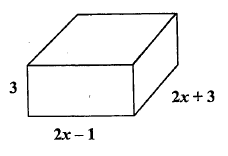1.5 The student demonstrates partial knowledge of the simpler details and processes (Score 2.0 content) but exhibits major errors or omissions regarding the more complex ideas and procedures (Score 3.0 content).

1.0

(beginning)

With help, the student demonstrates a partial understanding of some of the simpler details and processes (Score 2.0 content) and some of the more complex ideas and processes (Score 3.0 content).
0.5 With help, the student demonstrates a partial understanding of some of the simpler details and processes (Score 2.0 content) but not the more complex ideas and processes (Score 3.0 content).
0.0 Even with help, the student demonstrates no understanding or skill.

## Resources

 Web Vocab

#### MAT-HS.A-CED.01.f

 MAT-HS Targeted Standards(A) Concept: Algebra(CED) Domain: Creating ExpressionsCluster: Create equations that describe numbers or relationships MAT-HS.A-CED.01.e Create simple rational equations

• I can

• I can

• I can

• I can

## Proficiency Scale

Score   Description Sample Activity

4.0

In addition to Score 3.0, the student demonstrates in-depth inferences and applications regarding more complex material that go beyond end of instruction expectations.
3.5 In addition to Score 3.0 performance, the student demonstrates in-depth inferences and applications regarding the more complex content with partial success.

3.0

(proficient)

The student can:
• create simple rational equations in one variable and use them to solve problems.
The student exhibits no major errors or omissions.

 Source  Illustrative Math: Basketball Chase and his brother like to play basketball. About a month ago they decided to keep track of how many games they have each won. As of today, Chase has won 18 out of the 30 games against his brother. How many games would Chase have to win in a row in order to have a 75% winning record?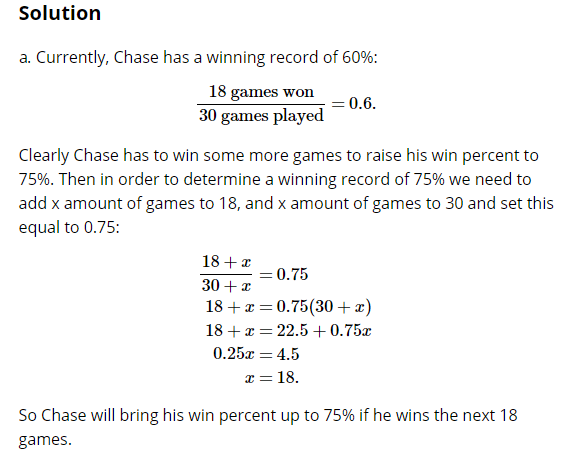2.5 The student demonstrates no major errors or omissions regarding the simpler details and processes (Score 2.0 content) and partial knowledge of the more complex ideas and processes (Score 3.0 content).

2.0

(progressing)

There are no major errors or omissions regarding the simpler details and processes as the student can:

• recognize and recall specific terminology such as:
• Solution
• Equation
• Rational Equation
• Inverse operations
• solve a linear equation.
• solve a simple rational equation.
However, the student exhibits major errors or omissions regarding the more complex ideas and processes.

Solve: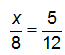Solve: 0.25(x+10)=14

1.5 The student demonstrates partial knowledge of the simpler details and processes (Score 2.0 content) but exhibits major errors or omissions regarding the more complex ideas and procedures (Score 3.0 content).

1.0

(beginning)

With help, the student demonstrates a partial understanding of some of the simpler details and processes (Score 2.0 content) and some of the more complex ideas and processes (Score 3.0 content). -
0.5 With help, the student demonstrates a partial understanding of some of the simpler details and processes (Score 2.0 content) but not the more complex ideas and processes (Score 3.0 content).
0.0 Even with help, the student demonstrates no understanding or skill. -

## Resources

 Web Vocab

#### MAT-HS.A-CED.01.g

 MAT-HS Targeted Standards(A) Concept: Algebra(CED) Domain: Creating ExpressionsCluster: Create equations that describe numbers or relationships MAT-HS.A-CED.01.g Create exponential equations

• I can

• I can

• I can

• I can

## Proficiency Scale

 Score Description Sample Activity 4.0 (advanced) In addition to Score 3.0, the student demonstrates in-depth inferences and applications regarding more complex material that go beyond end of instruction expectations. 3.5 In addition to Score 3.0 performance, the student demonstrates in-depth inferences and applications regarding the more complex content with partial success. 3.0 (proficient) The student can: create exponential equations in one variable and use them to solve problems. The student exhibits no major errors or omissions. During normal breathing, about 12% of the air in the lungs is replaced after one breath. Write an exponential decay model for the amount of the original air left in the lungs if the initial amount of air in the lungs is 500 mL. How much of the original air is present after 240 breaths? The foundation of your house has about 1,200 termites. The termites grow at a rate of about 2.4% per day. How long until the number of termites doubles? 2.5 The student demonstrates no major errors or omissions regarding the simpler details and processes (Score 2.0 content) and partial knowledge of the more complex ideas and processes (Score 3.0 content). 2.0 (progressing) There are no major errors or omissions regarding the simpler details and processes as the student can: recognize and recall specific terminology such as: Exponential Decay Growth Rate Equation Annually Semi-Annually Quarterly Compounded create the equation; however, is unable to solve. solve a quadratic equation. However, the student exhibits major errors or omissions regarding the more complex ideas and processes. Solve: 50 = 2(3)x 1.5 The student demonstrates partial knowledge of the simpler details and processes (Score 2.0 content) but exhibits major errors or omissions regarding the more complex ideas and procedures (Score 3.0 content). 1.0 (beginning) With help, the student demonstrates a partial understanding of some of the simpler details and processes (Score 2.0 content) and some of the more complex ideas and processes (Score 3.0 content). 0.5 With help, the student demonstrates a partial understanding of some of the simpler details and processes (Score 2.0 content) but not the more complex ideas and processes (Score 3.0 content). 0.0 Even with help, the student demonstrates no understanding or skill.

## Resources

 Web Vocab

#### MAT-HS.A-CED.02

 MAT-HS Targeted Standards(A) Concept: Algebra(CED) Domain: Creating ExpressionsCluster: Create equations that describe numbers or relationships MAT-HS.A-CED.02 Create equations in two or more variables to represent relationships between quantities; graph equations on coordinate axes with labels and scales.

This standard is divided into parts.  Click on these links to see specific proficiency scales.

MAT-HS.A-CED.02.a

MAT-HS.A-CED.02.b

#### MAT-HS.A-CED.02.a

 MAT-HS Targeted Standards(A) Concept: Algebra(CED) Domain: Creating ExpressionsCluster: Create equations that describe numbers or relationships MAT-HS.A-CED.02.a Create equations in two or more variables to represent relationships between quantities; graph equations on coordinate axes with labels and scales.

## Student Learning Targets:

• I can
• I can

• I can
• I can

### Skills (Performance) Targets

• I can create an equation in two or more variables to represent relationships between quantities.
• I can graph equations in two variables on a coordinate plane and label the axes and scales.

• I can

## Proficiency Scale

 Score Description Sample Activity 4.0 In addition to achieving level 3.0 content, the student makes in-depth inferences and applications that go beyond what was taught including construct linear functions, including arithmetic and geometric sequences, given a graph, a description of a relationship, or two input-output pairs (A.F.LE.2) - 3.5 In addition to Score 3.0 performance, the student demonstrates in-depth inferences and applications regarding the more complex content with partial success. 3.0 The student can: create linear equations in two or more variables to represent relationships between quantities   graph equations on coordinate axes with labels and scales The student exhibits no major errors or omissions. Suppose $15 US is worth$150 Mexican pesos. What is an equation that relates US dollars x to Mexican pesos y? The cost to rent a car is $50 plus$0.25 per mile driven.  Write and graph an equation to represent the situation. 2.5 The student demonstrates no major errors or omissions regarding the simpler details and processes (Score 2.0 content) and partial knowledge of the more complex ideas and processes (Score 3.0 content). 2.0 There are no major errors or omissions regarding the simpler details and processes as the student: recognizes and recalls specific terminology, such as:  (A.SSE.1)                linear However, the student exhibits major errors or omissions regarding the more complex ideas and processes. - 1.5 The student demonstrates partial knowledge of the simpler details and processes (Score 2.0 content) but exhibits major errors or omissions regarding the more complex ideas and procedures (Score 3.0 content). 1.0 With help, a partial understanding of some of the simpler details and processes and some of the more complex ideas and processes. - 0.5 With help, the student demonstrates a partial understanding of some of the simpler details and processes (Score 2.0 content) but not the more complex ideas and processes (Score 3.0 content).

## Alg II Create and Graph Quadratic Functions Proficiency Scale

 Score Description Sample Activity 4.0 (advanced) In addition to Score 3.0, the student demonstrates in-depth inferences and applications regarding more complex material that go beyond end of instruction expectations. Given two parabolic graphs, create the equations for both functions, graph them, and describe the similarities and differences. Function 1:  the turning point is (2,-3) and another random point on the graph is (4,5)   Function 2:  the turning point is (2,-3) and another random point on the graph is (5,6) 3.5 In addition to Score 3.0 performance, the student demonstrates in-depth inferences and applications regarding the more complex content with partial success. 3.0 (proficient) The student can: create quadratic equations in two variables given information about the function. The student exhibits no major errors or omissions. Given the x-intercepts of 6 and -2, write a quadratic function that would also pass through the point (1,5). 2.5 The student demonstrates no major errors or omissions regarding the simpler details and processes (Score 2.0 content) and partial knowledge of the more complex ideas and processes (Score 3.0 content). 2.0 (progressing) There are no major errors or omissions regarding the simpler details and processes as the student can: recognize and recall specific terminology such as: quadratic identify the graph and/or equation of quadratic functions two variables on a coordinate plane and label the axes and scales. However, the student exhibits major errors or omissions regarding the more complex ideas and processes. Given the graph below, write the quadratic function.1.5 The student demonstrates partial knowledge of the simpler details and processes (Score 2.0 content) but exhibits major errors or omissions regarding the more complex ideas and procedures (Score 3.0 content). 1.0 (beginning) With help, the student demonstrates a partial understanding of some of the simpler details and processes (Score 2.0 content) and some of the more complex ideas and processes (Score 3.0 content). 0.5 With help, the student demonstrates a partial understanding of some of the simpler details and processes (Score 2.0 content) but not the more complex ideas and processes (Score 3.0 content). 0.0 Even with help, the student demonstrates no understanding or skill.

## Alg II Create and Graph Exponential Functions Proficiency Scale

 Score Description Sample Activity 4.0 (advanced) In addition to Score 3.0, the student demonstrates in-depth inferences and applications regarding more complex material that go beyond end of instruction expectations. Given two exponential graphs, create the equations for both functions, graph them, and describe the similarities and differences.Function 1:  contains the point (0, 4) and (1, 12)   Function 2:  contains the point (0, 4) and (1, 2) 3.5 In addition to Score 3.0 performance, the student demonstrates in-depth inferences and applications regarding the more complex content with partial success. 3.0 (proficient) The student can: create exponential equations in two variables given information about the function. The student exhibits no major errors or omissions. Given the points (0, 3) and (-1, 6), create the exponential equation. 2.5 The student demonstrates no major errors or omissions regarding the simpler details and processes (Score 2.0 content) and partial knowledge of the more complex ideas and processes (Score 3.0 content). 2.0 (progressing) There are no major errors or omissions regarding the simpler details and processes as the student can: recognize and recall specific terminology such as: exponential identify the graph and/or equation of exponential functions in two variables on a coordinate plane and label the axes and scales. However, the student exhibits major errors or omissions regarding the more complex ideas and processes. Given the graph below, write the exponential function.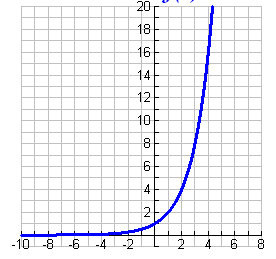1.5 The student demonstrates partial knowledge of the simpler details and processes (Score 2.0 content) but exhibits major errors or omissions regarding the more complex ideas and procedures (Score 3.0 content). 1.0 (beginning) With help, the student demonstrates a partial understanding of some of the simpler details and processes (Score 2.0 content) and some of the more complex ideas and processes (Score 3.0 content). - 0.5 With help, the student demonstrates a partial understanding of some of the simpler details and processes (Score 2.0 content) but not the more complex ideas and processes (Score 3.0 content). 0.0 Even with help, the student demonstrates no understanding or skill. -

## Alg II Create and Graph Polynomial Functions Proficiency Scale

 Score Description Sample Activity 4.0 (advanced) In addition to Score 3.0, the student demonstrates in-depth inferences and applications regarding more complex material that go beyond end of instruction expectations. Given two polynomial graphs, create the equations for both functions, graph them, and describe the similarities and differences. Function 1:  the zeros are  1, 2, 3, and another random point on the graph is (0,-6)   Function 2:  the zeros are 1, 2,3, and another random point on the graph is (0,18) 3.5 In addition to Score 3.0 performance, the student demonstrates in-depth inferences and applications regarding the more complex content with partial success. 3.0 (proficient) The student can: create polynomial equations in two variables on a coordinate plane and label the axes and scales. The student exhibits no major errors or omissions. Given the x-intercepts of -4, -1 and 2, write a polynomial function that would also pass through the point (0,-2). 2.5 The student demonstrates no major errors or omissions regarding the simpler details and processes (Score 2.0 content) and partial knowledge of the more complex ideas and processes (Score 3.0 content). 2.0 (progressing) There are no major errors or omissions regarding the simpler details and processes as the student can: recognize and recall specific terminology such as: polynomial identify the graph and/or equation of polynomial functions in two variables on a coordinate plane and label the axes and scales.    However, the student exhibits major errors or omissions regarding the more complex ideas and processes. Given the graph below, write the polynomial function.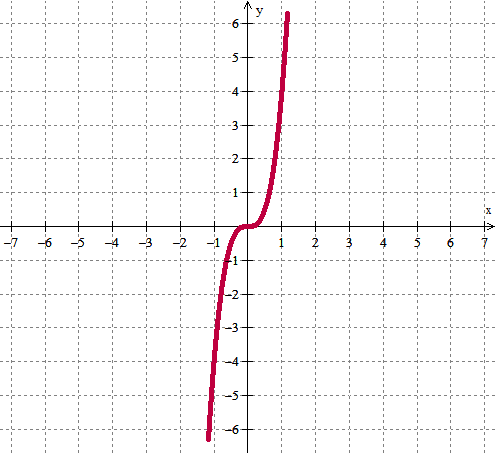1.5 The student demonstrates partial knowledge of the simpler details and processes (Score 2.0 content) but exhibits major errors or omissions regarding the more complex ideas and procedures (Score 3.0 content). 1.0 (beginning) With help, the student demonstrates a partial understanding of some of the simpler details and processes (Score 2.0 content) and some of the more complex ideas and processes (Score 3.0 content). - 0.5 With help, the student demonstrates a partial understanding of some of the simpler details and processes (Score 2.0 content) but not the more complex ideas and processes (Score 3.0 content). 0.0 Even with help, the student demonstrates no understanding or skill. -

## Resources

 Web Vocab coordinate plane scale

#### MAT-HS.A-CED.03

 MAT-HS Targeted Standards(A) Concept: Algebra(CED) Domain: Creating ExpressionsCluster: Create equations that describe numbers or relationships MAT-HS.A-CED.03* Represent constraints by equations or inequalities, and by systems of equations and/or inequalities, and interpret solutions as viable or nonviable options in a modeling context. For example, represent inequalities describing nutritional and cost constraints on combinations of different foods.

## Student Learning Targets:

• I can
• I can

• I can
• I can

### Skills (Performance) Targets

• I can write and use a system of equations and/or inequalities to solve a real world problem.
• I can use equations and inequalities to represent problem constraints and objectives (linear programming).

• I can
• I can

## Resources

 Web Vocab linear programming

Page: (Previous)   1  2  3  4  5  (Next)
ALL